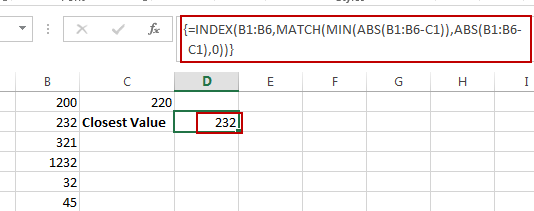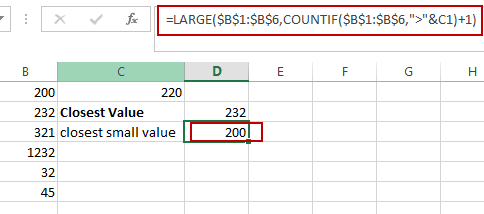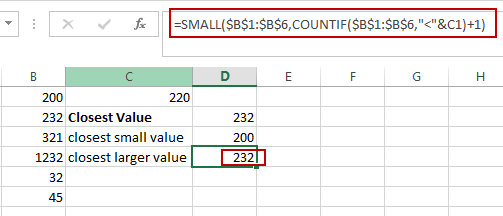# Find Closest Value or Nearest Value in a Range in Excel

This post will guide you how to find the closest value or nearest value in a range of cells or in a column in Excel. How do I find the closest match in a range to a given lookup value in Excel. How to find the closest larger and closest smaller values from a range of cells in excel.

## Find Closest Value or Nearest Value in a Range

Assuming that you have a list of numbers in range of cells B1:B6, and you have a lookup value in Cell C1, and you want to find the nearest value that matches the lookup value in that range. How to achieve it.

You need to use an excel array formula based on the INDEX function, the MATCH function, the MIN function and the ABS function. Just like this:

`=INDEX(B1:B6,MATCH(MIN(ABS(B1:B6-C1)),ABS(B1:B6-C1),0))`

Type this formula into a blank cell D2, and press CTRL+SHIFT+Enter keys in your keyboard. and the nearest value is retrieved from the range B1:B6.## Find Closest Smaller Value

If you want to find the closest smaller value from a range of cells in excel, you need to use another formula based on the LARGE function and the COUNTIF function. Just like this:

`=LARGE(\$B\$1:\$B\$6,COUNTIF(\$B\$1:\$B\$6,">"&C1)+1)`

Type this formula into a blank cell, and then press Enter key. and the closest smallest value will be retrieved from the given range of cells.## Find Closest Largest Value

If you want to find the closest largest value from a range of cells in excel, you need to use another formula based on the SMALL function and  the COUNTIF function. Just like this:

`=SMALL(\$B\$1:\$B\$6,COUNTIF(\$B\$1:\$B\$6,”<”&C1)+1)`

Type this formula into a blank cell, and then press Enter key. and the closest largest value will be retrieved from the given range of cells.### Related Functions

• Excel COUNTIF function
The Excel COUNTIF function will count the number of cells in a range that meet a given criteria. This function can be used to count the different kinds of cells with number, date, text values, blank, non-blanks, or containing specific characters.etc.= COUNTIF (range, criteria)…
• Excel INDEX function
The Excel INDEX function returns a value from a table based on the index (row number and column number)The INDEX function is a build-in function in Microsoft Excel and it is categorized as a Lookup and Reference Function.The syntax of the INDEX function is as below:= INDEX (array, row_num,[column_num])…
• Excel MATCH function
The Excel MATCH function search a value in an array and returns the position of that item.The syntax of the MATCH function is as below:= MATCH  (lookup_value, lookup_array, [match_type])….
• Excel SMALL function
The Excel SMALL function returns the smallest numeric value from the numbers that you provided. Or returns the smallest value in the array.The syntax of the SMALL function is as below:=SMALL(array,nth) …
• Excel MIN function
The Excel MIN function returns the smallest numeric value from the numbers that you provided. Or returns the smallest value in the array.The MIN function is a build-in function in Microsoft Excel and it is categorized as a Statistical Function.The syntax of the MIN function is as below:= MIN(num1,[num2,…numn])….
• Excel LARGE function
The Excel LARGE function returns the largest numeric value from the numbers that you provided. Or returns the largest value in the array. The syntax of the LARGE function is as below:= LARGE (array,nth)…
• Excel ABS Function
The Excel ABS function returns the absolute value of a number.The ABS function is a build-in function in Microsoft Excel and it is categorized as a Math and Trigonometry Function.The syntax of the ABS function is as below:=ABS (number)…